# Invariance, principle of

(diff) ← Older revision | Latest revision (diff) | Newer revision → (diff)

Letbe independent identically-distributed real-valued random variables with zero expectation and variance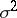; consider the random polygonal line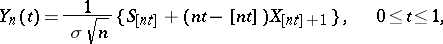where. Ifis a real-valued continuous function on the space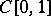of continuous functions onwith the supremum norm (or only continuous everywhere except on a set of Wiener measure zero), then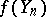converges in distribution to, whereis a Wiener random function. Thus, the limiting distribution for thedoes not depend on any special properties of the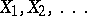.

A typical scheme for the use of the invariance principle consists in finding the limiting distribution for theby finding the limiting distribution for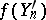, where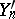is a random polygonal line constructed in the same way as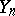from some specially chosen sequence. For example, ifthenis continuous on, and, since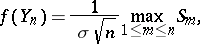one has that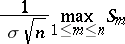converges in distribution to. To find the distribution of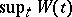, the sequence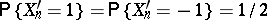is used, and as a result of the calculations one obtains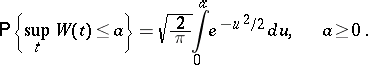How to Cite This Entry:
Invariance, principle of. Encyclopedia of Mathematics. URL: http://encyclopediaofmath.org/index.php?title=Invariance,_principle_of&oldid=13150
This article was adapted from an original article by V.V. Sazonov (originator), which appeared in Encyclopedia of Mathematics - ISBN 1402006098. See original article